# 1. Contin 1. Crickets make a chirping noise by sliding their wings rapidly over each other....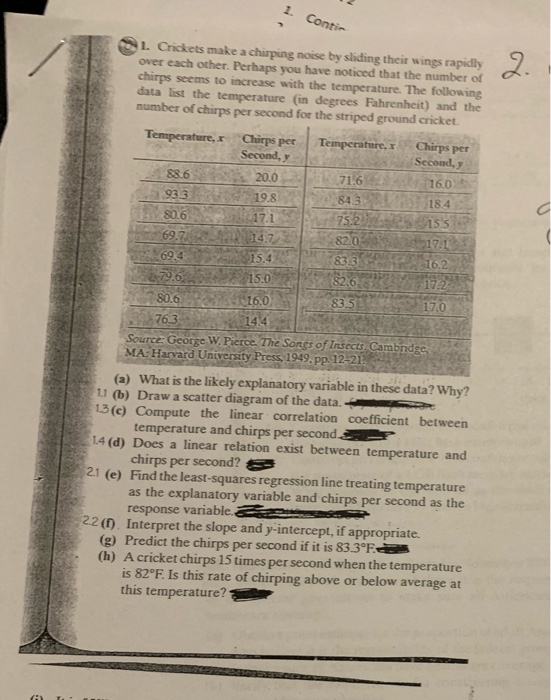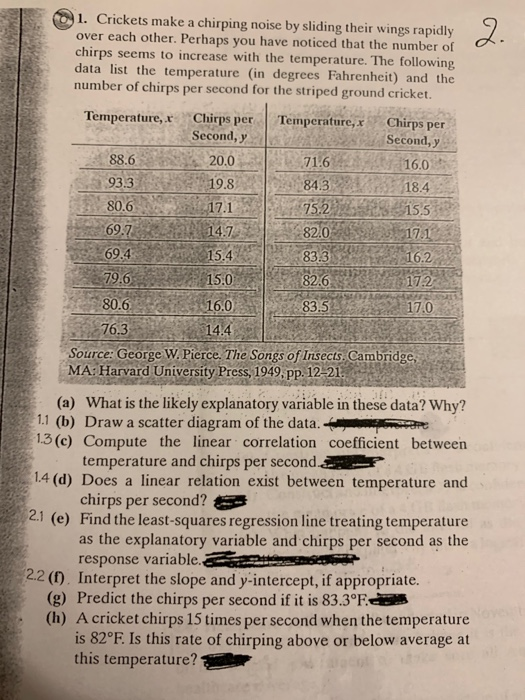1. Contin 1. Crickets make a chirping noise by sliding their wings rapidly over each other. Perhaps you have noticed that the number of chirps seems to increase with the temperature. The following data list the temperature (in degrees Fahrenheit) and the number of chirps per second for the striped ground cricket. Temperature, Chirps per Temperature, Chirps per Second, Second, 88.6 20.0 16.0 933 716 79.6 80. 6 16. 01 153 17.0 76. 3 144 Source: George W. Pierce. The Songs of Insects. Cambridge MA: Harvard University Press, 1949. pp. 12-21 (a) What is the likely explanatory variable in these data? Why? 11 (b) Draw a scatter diagram of the data. 13C) Compute the linear correlation coefficient between temperature and chirps per seconds 14 (d) Does a linear relation exist between temperature and chirps per second? 21 (e) Find the least-squares regression line treating temperature as the explanatory variable and chirps per second as the response variablea 22 (1). Interpret the slope and y-intercept, if appropriate. (g) Predict the chirps per second if it is 83.3°F (h) A cricket chirps 15 times per second when the temperature is 82°F Is this rate of chirping above or below average at this temperature?
1. Crickets make a chirping noise by sliding their wings rapidly over each other. Perhaps you have noticed that the number of chirps seems to increase with the temperature. The following data list the temperature (in degrees Fahrenheit) and the number of chirps per second for the striped ground cricket. 1720 Temperature, Chirps per Temperature, x Chirps per Second, y Second, y 88.6 20.0 71.6 16.0 93.3 19.8 84.3 18.4 80.6 17.1 75. 2 15.5 69.7 14.7 82.0 17.1 69.4 15.4 83.3 16.2 79. 6 15.0 82.6 172 80.6. 0 16. 8 3.5 76.3 14.4 Source: George W. Pierce. The Songs of Insects. Cambridge, MA: Harvard University Press, 1949, pp. 12-21. (a) What is the likely explanatory variable in these data? Why? 1.1 (b) Draw a scatter diagram of the data. 1.3 (e) Compute the linear correlation coefficient between temperature and chirps per second.. 1.4 (d) Does a linear relation exist between temperature and chirps per second? 2.1 (e) Find the least-squares regression line treating temperature as the explanatory variable and chirps per second as the response variable. 2.2 (0). Interpret the slope and y-intercept, if appropriate. (g) Predict the chirps per second if it is 83.3°F. (h) A cricket chirps 15 times per second when the temperature is 82°F Is this rate of chirping above or below average at this temperature?

(a)

Temperature is explanatory variable because researcher wants to find the effect of temperature on the number of Chirps.

(b)

Following is the scatter plot: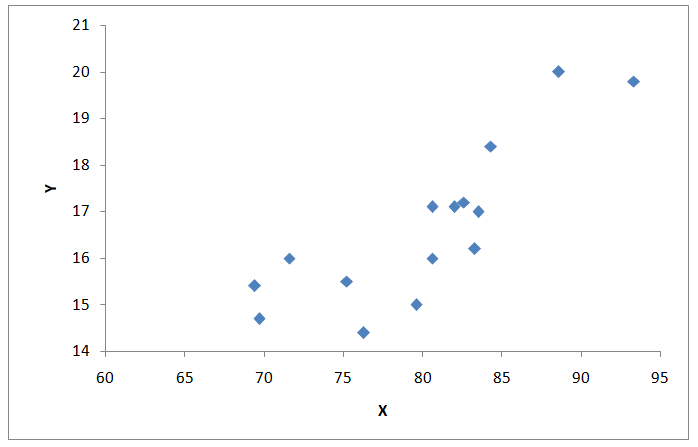(c)

Following table shows the calculations

 X Y X^2 Y^2 XY 88.6 20 7849.96 400 1772 93.3 19.8 8704.89 392.04 1847.34 80.6 17.1 6496.36 292.41 1378.26 69.7 14.7 4858.09 216.09 1024.59 69.4 15.4 4816.36 237.16 1068.76 79.6 15 6336.16 225 1194 80.6 16 6496.36 256 1289.6 76.3 14.4 5821.69 207.36 1098.72 71.6 16 5126.56 256 1145.6 84.3 18.4 7106.49 338.56 1551.12 75.2 15.5 5655.04 240.25 1165.6 82 17.1 6724 292.41 1402.2 83.3 16.2 6938.89 262.44 1349.46 82.6 17.2 6822.76 295.84 1420.72 83.5 17 6972.25 289 1419.5 Total 1200.6 249.8 96725.86 4200.56 20127.47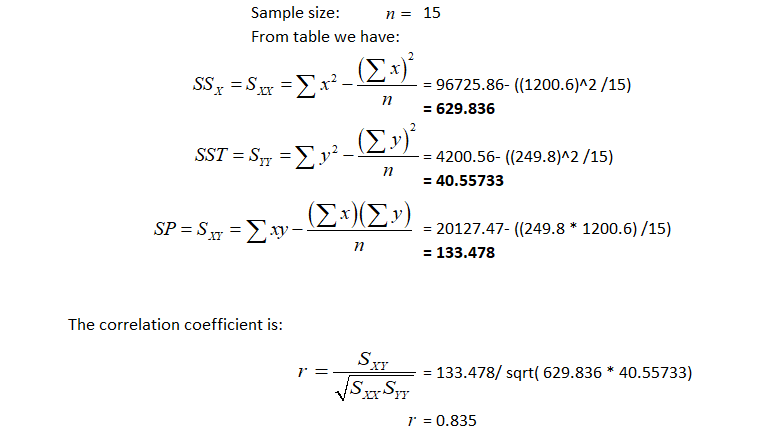(d)

The correlation coefficient between the variables is strong and positive. Scatter plot also shows a strong , positive linear relationship between the variables. So there exists a relationship between the variables.

(e)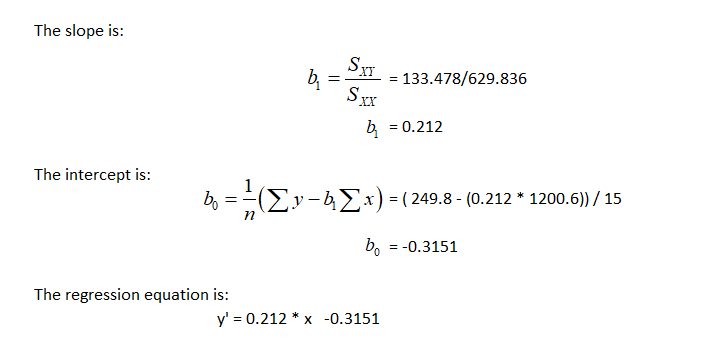(f)

Slope: It is 0.212. It shows that for each unit increase in temperature chirps increased by 0.212 units.

Intercept: It is -0.3151. When temperature is zero the number of chirps is -0.3151. It is not possible so it is meaningless in this case.

(g)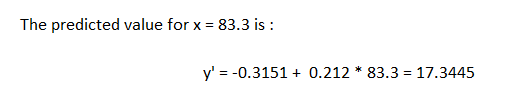(h)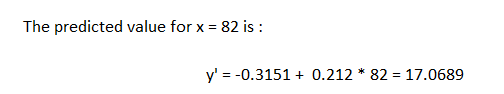The actual value 15 is lower than predicted value so it is below average.

##### Add Answer to: 1. Contin 1. Crickets make a chirping noise by sliding their wings rapidly over each other....
Similar Homework Help Questions
• ### ONLY A TO C ONLY A TO C ONLY A TO C ONLY A TO C...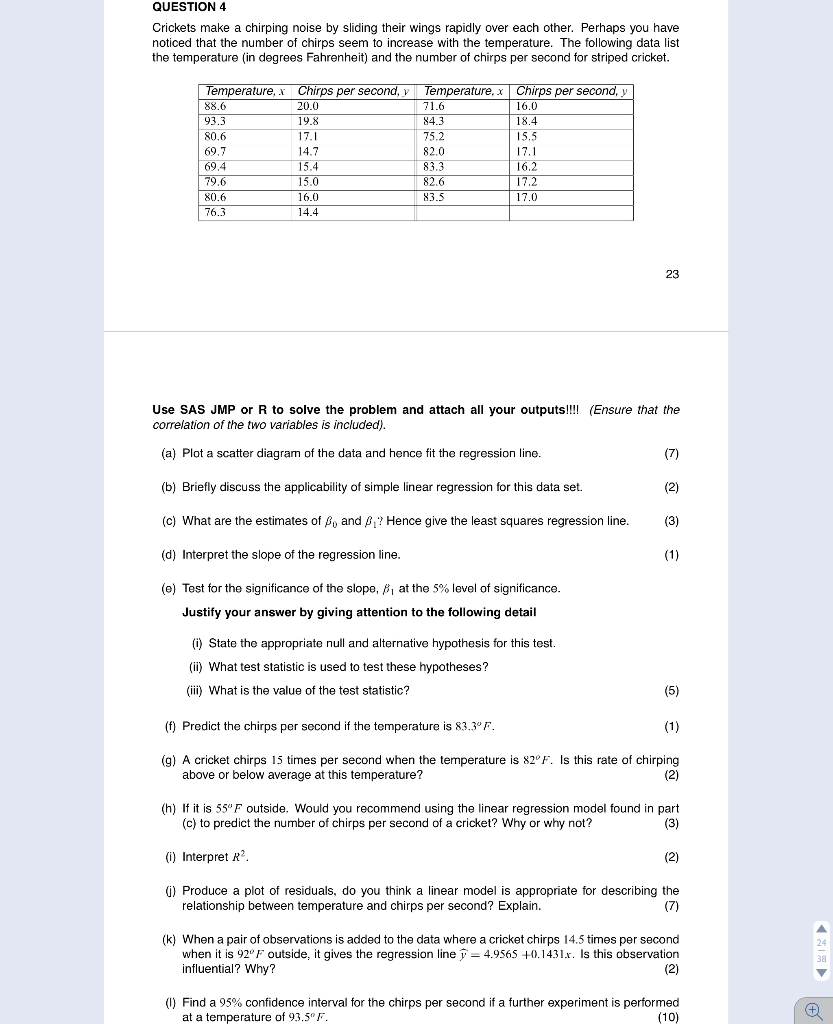ONLY A TO C ONLY A TO C ONLY A TO C ONLY A TO C QUESTION 4 Crickets make a chirping noise by sliding their wings rapidly over each other. Perhaps you have noticed that the number of chirps seem to increase with the temperature. The following data list the temperature (in degrees Fahrenheit) and the number of chirps per second for striped cricket. Temperature, .x Chirps per second, 88.6 20.0 93.3 19.8 80.6 17.1 69.7 14.7 69.4 15.4...

• ### A biologist wishes to determine if there is a relationship between temperature and the number of...

A biologist wishes to determine if there is a relationship between temperature and the number of chirps per second of crickets. Below is the data of 15 crickets at various temperatures. Chirps/sec Temperature 20 88.6 16 71.6 19.8 93.3 18.4 84.3 17.1 80.6 15.5 75.2 14.7 69.7 17.1 82 15.4 69.4 16.2 83.3 15 79.6 17.2 82.6 16 80.6 17 83.5 14.4 76.3 Then the linear formula is: Chirps =___+___ *Temperature

Free Homework App